# Math in Focus Grade 7 Chapter 8 Lesson 8.3 Answer Key Finding Volume and Surface Area of Pyramids and Cones

This handy Math in Focus Grade 7 Workbook Answer Key Chapter 8 Lesson 8.3 Finding Volume and Surface Area of Pyramids and Cones detailed solutions for the textbook questions.

## Math in Focus Grade 7 Course 2 B Chapter 8 Lesson 8.3 Answer Key Finding Volume and Surface Area of Pyramids and Cones

Hands-On Activity

Materials:

• nets for a pyramid, a triangular prism, a cylinder, and a cone
• scissors
• ruler
• tape
• rice grains

EXPLORE VOLUME RELATIONSHIPS BETWEEN A PYRAMID AND A PRISM, AND BETWEEN A CONE AND A CYLINDER

Work in pairs.

STEP 1: Cut out the nets for a triangular pyramid and triangular prism.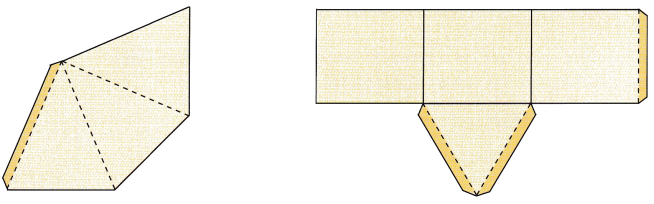STEP 2: Fold the nets to make a triangular pyramid and triangular prism. Each solid will be open at one end. Tape the edges to hold them in place.

STEP 3: Do the pyramid and prism have the same height? Do the base of the pyramid and the base of the prism have about the same area?

STEP 4: Fill the pyramid completely with rice grains and pour them into the prism. Keep doing this until the prism is filled with rice grains. Flow many pyramids of rice grains are needed to fill the prism?

Math Journal What is the relationship between the volume of a triangular pyramid and the volume of a triangular prism with the same base and height? Suggest a formula for the volume of a pyramid.

STEP 5: Cut out the nets for a cylinder and cone.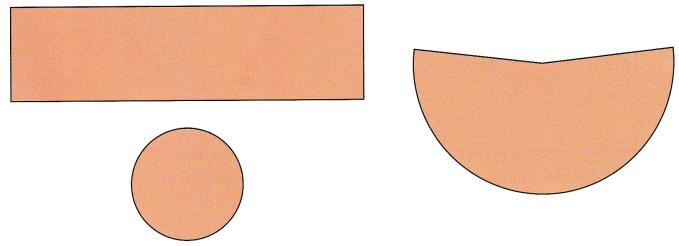STEP 6: Use the cutouts of the rectangle and circle to make a cylinder. Tape the edges to hold them in place. The cylinder will be open at one end.

STEP 7: Fold the cutout of the sector of the circle to make a cone. Tape the edges to hold them in place. The cone will be open at its wide end.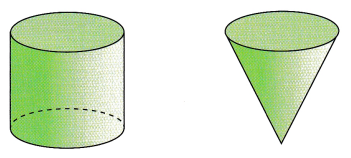STEP 8: Are the cylinder and the cone about the same height? Do the base of the cylinder and the base of the cone have about the same area?

STEP 9: Fill the cone completely with rice grains and pour them into the cylinder. Keep doing this until the cylinder is filled with rice grains. How many cones of rice grains are needed to fill the cylinder?

Math Journal What is the relationship between the volume of a cone and the volume of a cylinder with the same base and height? Suggest a formula for the volume of a cone.
Answer: The relationship between the volume of a cone and the volume of a cylinder with the same base and height is the volume of the cone is one-third the volume of the cylinder.

Explanation:
We know that the volume of the cylinder is  V = πr²h
the volume of the cone V=1/3hπr².
Then, The relationship between the volume of a cone and the volume of a cylinder,
with the same base and height is the volume of the cone is one-third the volume of the cylinder.
And, $$\frac{1}{3}$$ πr²h = $$\frac{1}{3}$$ πr²h
Hence, The volume of the cone and the volume of the cylinder is equal when their respective base and height are equal.

### Math in Focus Grade 7 Chapter 8 Lesson 8.3 Guided Practice Answer Key

Solve.

Question 1.
The base of a pyramid is a right triangle. The triangle has a base of 5 centimeters and a height of 3 centimeters. The pyramid has a height of 6 centimeters. What is the volume of the pyramid?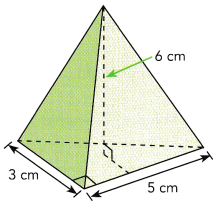Use the formula for the volume of a pyramid.
Volume of pyramid
= $$\frac{1}{3}$$ Bh Use the formula.
= $$\frac{1}{3}$$ ∙ (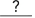) ∙Substitute for B and h.
=cm3 Multiply.
The volume of the pyramid iscubic centimeters.
Answer:  The volume of the pyramid is 10 cm³

Explanation:
The volume of the pyramid is $$\frac{1}{3}$$ bh
Here b = 5 cm, h = 6 cm
V = $$\frac{1}{3}$$ ($$\frac{1}{2}$$ × 3 × 5) 6
V = 15 cm³
So, The volume of the pyramid is 15 cm³.

Question 2.
A square pyramid has a height of 12 meters. If a side of the base measures 5.5 meters, what is the volume of the pyramid?
Answer: The volume of the Square pyramid is 121 cm³.

Explanation:
The volume of the Square pyramid is $$\frac{1}{3}$$ a²h
Here a = 5.5 m, h = 12 m
V = $$\frac{1}{3}$$ (5.5 × 5.5 × 12)
= 30.25 × 4
V = 121 m³
So, The volume of the Square pyramid is 121 cm³.

Question 3.
A square pyramid has a volume of 400 cubic centimeters. The length of the base is 10 centimeters. What is the height of the pyramid?
Let the height of the pyramid be h centimeters.
Volume of pyramid = $$\frac{1}{3}$$ Bh Use the formula.= $$\frac{1}{3}$$ ∙ () ∙ h Substitute for the volume and dimensions of the base.=∙ h Multiply both sides by.=Divide both sides by= h Simplify.
The height of the pyramid iscentimeters.
Answer: The height of the Square pyramid is 12 cm.

Explanation:
The volume of the Square pyramid is 400 cm³
Here a = 10 cm, h = ?
V = $$\frac{1}{3}$$ a²h
400 = $$\frac{1}{3}$$ 10 × 10 × h
h = $$\frac{400 × 3}{10 × 10}$$
= 12 cm
So, The height of the Square pyramid is 12 cm.

Question 4.
A rectangular pyramid has a volume of 58 cubic meters. If the sides of the base measure 3 meters by 5 meters, what is the height of the pyramid?
Answer: The height of the Rectangular pyramid is 11.6 m.

Explanation:
The volume of the Rectangular pyramid is 58 m³
Here l = 5 m, b = 3 m , h = ?
V = $$\frac{1}{3}$$ lbh
58 = $$\frac{1}{3}$$ 5 × 3 × h
h = $$\frac{58 × 3}{5 × 3}$$
= 11.6 m
So, The height of the Rectangular pyramid is 11.6 m.

Question 5.
A party hat is in the shape of a cone. Find the exact volume of the party hat. Use 3.14 as an approximation for π to find the approximate volume of the cone. Round your answer to the nearest tenth.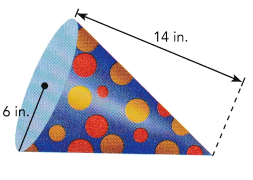Use the formula for the volume of a cone, V = $$\frac{1}{3}$$Bh
Volume of the party hat = $$\frac{1}{3}$$ ∙ πr2 ∙ h Use the formula.
= $$\frac{1}{3}$$ ∙Substitute for π, r, and h.
=Multiply.in3 Round to the nearest tenth.
The exact volume of the party hat iscubic inches.
An approximate volume iscubic inches.
Answer: The volume of the party hat is 504 in³.

Explanation:
the volume of the cone V=1/3hπr².
Here r = 6 in. , h = 14 in.
V = $$\frac{1}{3}$$ 14 × 6 × 6 × 3.14
V = 504 in³.
So, The volume of the party hat is 504 in³.

Question 6.
The diagram shows a cone-shaped container. Find the exact volume of the container. Use 3.14 as an approximation for π to find the approximate volume of the container. Round your answer to the nearest tenth.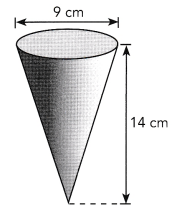Answer: The volume of the container is 283 cm³.

Explanation:
the volume of the cone V=1/3hπr².
Here d= 9 cm , then
r = 4.5 cm , h = 14 cm.
V = $$\frac{1}{3}$$ 14 × 4.5 × 4.5 × 3.14
V = 283.5 cm³.
So, The volume of the container is 283 cm³.

Question 7.
A cone has a height of 57 centimeters and a volume of 2,923.34 cubic centimeters. What is the radius of the cone? Use 3.14 as an approximation for π.
Let the height of the cone be h centimeters.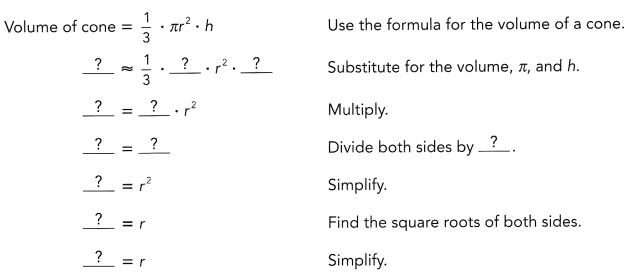The radius of the cone is aboutcentimeters.

Explanation:
the volume of the cone V=1/3hπr².
Here V = 2923.34 cm³
r = ? , h = 57 cm.
2923.34 = $$\frac{1}{3}$$ 57 × r² × 3.14
r² = $$\frac{2923.34 × 3}{57 × 3.14}$$
r² =51.28 cm.
r = 7.16 cm
So, The radius of the cone  is 7.16 cm.

Question 8.
A cone has a height of 7.2 inches and a volume of 188.4 cubic inches. What is the radius of the cone? Use 3.14 as an approximation for π.

Explanation:
the volume of the cone V=1/3hπr².
Here V = 188.4 in³
r = ? , h =7.2 in.
188.4 = $$\frac{1}{3}$$ 7.2 × r² × 3.14
r² = $$\frac{188.4 × 3}{7.2 × 3.14}$$
r² =26.16 in.
r = 5.11 in
So, The radius of the cone  is 5.11 in.

Question 9.
Jean wants to sell lemonade in cone-shaped paper cups. Each cup has a diameter of 4 centimeters and a height of 8 centimeters. She wants to make enough lemonade for 50 cups. How much lemonade does Jean need to make? Round your answer to the nearest tenth. Use 3.14 as an approximation for π.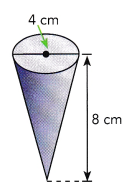Explanation:
the volume of the cone V=1/3hπr².
Here d= 4 cm , then
r = 2 cm , h = 8 cm.
V = $$\frac{1}{3}$$ 8 × 2 × 2 × 3.14
V = 32 cm³.
So, The volume of the cup is 32 cm³.

Given, She wants to make enough lemonade for 50 cups.
= 50 × 32
= 1600 ml

Hands-On Activity

FIND A FORMULA FOR THE AREA OF THE CURVED SURFACE OF A CONE

Work in pairs.

STEP 1: Copy and complete the table. For each pyramid, the base has edges of length b. The slant height is h.STEP 2: In general, what is the formula for the area of all the lateral triangular faces of a pyramid?

STEP 3: Suppose the number of sides of the base of the pyramid increases as shown below. Name the solid that is eventually formed.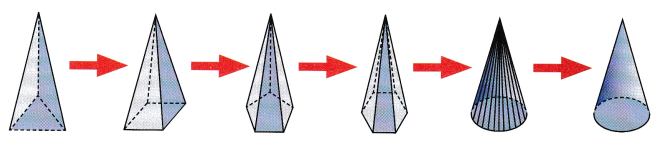STEP 4: The formula for the curved surface area of a cone is related to the formula for the surface area of the triangular faces of a pyramid. Copy and complete the formula for the curved surface area of a cone. Let r represent the radius of the base of a cone. Let € represent the slant height of the cone.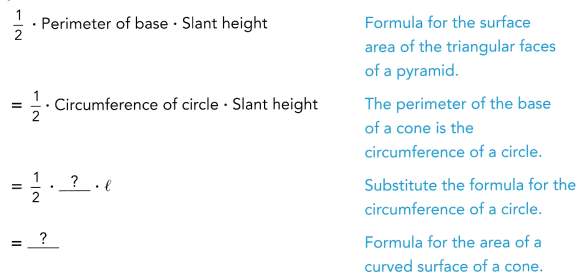Question 10.
A solid cone has a radius of 7 inches and a slant height of 14 inches.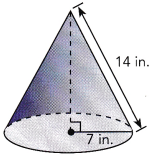a) What is the exact area of the cone’s curved surface?
πrl =Use the formula for the lateral surface area of a cone.
=in2 Multiply.
The exact area of the cone’s curved surface issquare inches.
Answer: The exact area of the cone’s curved surface is 307.72 in²

Explanation:
The curved surface area of a cone  S =  πrl
Here r = 7 in, l = 14 in
S = 3.14 × 7 × 14
S = 307.72 in²
So, The exact area of the cone’s curved surface is 307.72 in²

b) What is the total surface area of the cone? Find both the exact value and an approximate value. Use $$\frac{22}{7}$$ an approximation for π.
Total surface area of the cone:
Area of base + Area of curved surface = πr2 +The lateral surface area is.
=+Substitutefor r.
=Multiply.
=Add.
=in2 Substitutefor π.
The total surface area of the cone is exactlysquare inches, and approximatelysquare inches.
Answer: The total surface area of the cone is exactly 462 in²

Explanation:
The total surface area of the cone is T = πr(r +l)
Here r = 7 in, l = 14 in
T = $$\frac{22}{7}$$ × 7 ( 7 + 14 )
= 21 × 22
T = 462 in²
So, The total surface area of the cone is exactly 462 in²

Question 11.
The radius of a cone is 3 inches, and the slant height is 12 inches.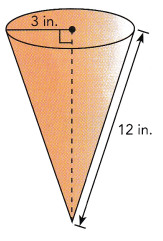a) What is the exact area of the cone’s curved surface?
Answer: The exact area of the cone’s curved surface is 113.04 in²

Explanation:
The curved surface area of a cone  S =  πrl
Here r = 3 in, l = 12 in
S = 3.14 × 3 × 12
S = 113.04 in²
So, The exact area of the cone’s curved surface is 113.04 in²

b) What is the total surface area of the cone? Find both the exact value and an approximate value. Use 3.14 as an approximation for π.
Answer: The total surface area of the cone is exactly 141.3 in²

Explanation:
The total surface area of the cone is T = πr(r +l)
Here r = 3 in, l = 12 in
T = 3.14 × 3 ( 3 + 12 )
= 3.14 × 45
T = 141.3 in²
So, The total surface area of the cone is exactly 141.3 in²

Question 12.
A conical straw hat has a diameter of 16 inches and a lateral surface area of 251.2 square inches. Find the approximate slant height of the straw hat. Use 3.14 as an approximation for π.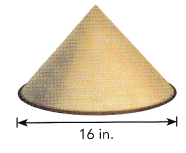Let the lateral height of the hat be l inches.
Area of lateral surface of hat = πrl Use the formula for the lateral surface area of a cone.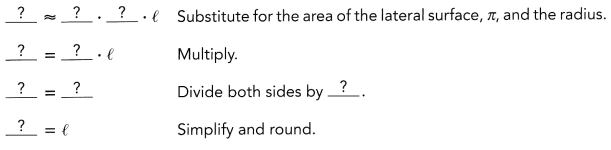The slant height of the hat is aboutinches.

Explanation:
Area of lateral surface of hat = πrl
Here, d = 16 in , S = 251.2 in²
Then r = 8 in
251.2 = 3.14 × 8 × l
l = $$\frac{251.2}{3.14 × 8}$$
l = 10 in
So, The slant height of the hat is about 10 in.

Question 13.
Jessica makes a cone-shaped paper filter to line a cone-shaped funnel. The funnel has a radius of 5 inches and a slant height of 10 inches. Suppose Jessica wants to make cone-shaped filters for 25 such funnels. About how many square inches of filter paper will she need? Use 3.14 as an approximation for π.
Answer: The filter paper she needs is  3925 in²

Explanation:
The lateral surface area of the funnel S = πrl
Here r = 5 in, l = 10 in
S = 3.14 × 5 × 10
S = 157 in²
Given, Jessica wants to make cone-shaped filters for 25 such funnels.
157 × 25 = 3925 in²
So, The filter paper she needs is  3925 in²

### Math in Focus Course 2B Practice 8.3 Answer Key

For this practice, you may use a calculator and use 3.14 as an approximation for π. Round your answers to the nearest tenth when you can.

Find the volume of each pyramid.

Question 1.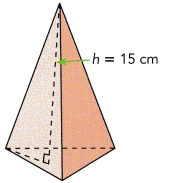Base area = 72 cm2
Answer:  The volume of the pyramid is 48 cm²

Explanation:
The volume of a pyramid is V = $$\frac{1}{3}$$bh
Given, h = 15 cm, A = 72 cm²
A = $$\frac{1}{2}$$bh
b = $$\frac{72 × 2}{15}$$
b = 9.6 cm

V = $$\frac{1}{3}$$ 9.6 × 15
V = 48cm²
So, The volume of the pyramid is 48 cm²

Question 2.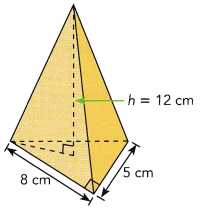Answer: The volume of the pyramid is 32 cm³.

Explanation:
The volume of the pyramid is $$\frac{1}{3}$$bh
Here b = 8 cm, h = 12 cm
V = $$\frac{1}{3}$$ 8 × 12
= 8 × 4
V = 32 cm³
So, The volume of the pyramid is 32 cm³.

Question 3.
A rectangular pyramid with a height of 10 inches and a base that measures 9 inches by 7 inches.
Answer: The volume of the rectangular  pyramid is 210 in³.

Explanation:
The volume of the rectangular pyramid is $$\frac{1}{3}$$lbh
Here b = 7 in, h = 10 in, l = 9 in
V = $$\frac{1}{3}$$ 9 × 7 × 10
= 30 × 7
V = 210 in³
So, The volume of the rectangular  pyramid is 210 in³.

Question 4.
A square pyramid with a height of 18 feet and a base that is 12 feet on each edge.
Answer: The volume of the square  pyramid is 864 ft³.

Explanation:
The volume of the square pyramid is $$\frac{1}{3}$$a²h
Here h = 18 ft, l = 12 ft
V = $$\frac{1}{3}$$ 12 × 12 × 18
= 144 × 6
V =864 ft³
So, The volume of the square  pyramid is 864 ft³.

Find the exact and approximate volume for each cone.

Question 5.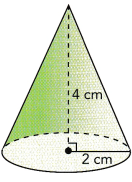Answer: The exact volume of the cone is 16.74 cm³.
The approximate volume of the cone is 16 cm³

Explanation:
the volume of the cone V=1/3hπr².
Here r = 2 cm. , h = 4 cm.
V = $$\frac{1}{3}$$ 4 × 2 × 2 × 3.14
V = 16.74 cm³.
So, The exact volume of the cone is 16.74 cm³.
The approximate volume of the cone is 16 cm³

Question 6.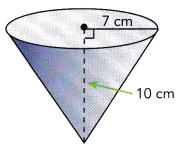Answer: The exact volume of the cone is 512.86 cm³.
The approximate volume of the cone is 512 cm³

Explanation:
the volume of the cone V=1/3hπr².
Here r = 7 cm. , h = 10 cm.
V = $$\frac{1}{3}$$ 10 × 7 × 7 × 3.14
V = 512.86 cm³.
So, The exact volume of the cone is 512.86 cm³.
The approximate volume of the cone is 512 cm³

Question 7.
A solid cone with a diameter of 10 centimeters and a height of 8 centimeters.
Answer: The exact volume of the cone is 209.33 cm³.
The approximate volume of the cone is 209 cm³

Explanation:
the volume of the cone V=1/3hπr².
Here, d = 10 cm,
r = 5 cm. , h = 8 cm.
V = $$\frac{1}{3}$$ 8 × 5 × 5 × 3.14
V = 209.33 cm³.
So, The exact volume of the cone is 209.33 cm³.
The approximate volume of the cone is 209 cm³

Question 8.
A cone with a radius of 4.9 centimeters and a height of 6.9 centimeters.
Answer:  The exact volume of the cone is 173.4 cm³.
The approximate volume of the cone is 173 cm³

Explanation:
the volume of the cone V=1/3hπr².
Here r = 4.9 cm. , h = 6.9 cm.
V = $$\frac{1}{3}$$ 6.9 × 4.9 × 4.9 × 3.14
V = 173.4 cm³.
So, The exact volume of the cone is 173.4 cm³.
The approximate volume of the cone is 173 cm³

Find the exact and an approximate surface area for each solid cone. Round your approximation to the nearest square unit.

Question 9.
A cone with a radius of 2.5 centimeters and a slant height of 5.6 centimeters.
Answer:  The exact area of the cone’s curved surface is 43.96 cm²
The  approximate area of the cone’s curved surface is 44 cm²

Explanation:
The curved surface area of a cone  S =  πrl
Here r = 2.5 cm , l = 5.6 cm
S = 3.14 × 2.5 × 5.6
S = 43.96 cm²
So, The exact area of the cone’s curved surface is 43.96 cm²
The  approximate area of the cone’s curved surface is 44 cm²

Question 10.
A cone with a diameter of 72 centimeters and a slant height of 48 centimeters.
Answer:  The exact area of the cone’s curved surface is 5425.92 cm²
The  approximate area of the cone’s curved surface is 5426 cm²

Explanation:
The curved surface area of a cone  S =  πrl
Here, d = 72 cm then,
r = 36 cm , l = 48 cm
S = 3.14 × 36 × 48
S = 5425.92 cm²
So, The exact area of the cone’s curved surface is 5425.92 cm²
The  approximate area of the cone’s curved surface is 5426 cm²

Question 11.
A cone with a diameter of 18 meters and a slant height of 22 meters.
Answer:  The exact area of the cone’s curved surface is 621.72 m²
The  approximate area of the cone’s curved surface is 622 m²

Explanation:
The curved surface area of a cone  S =  πrl
Here, d = 18 m then,
r = 9 m , l =  22 m
S = 3.14 × 9 × 22
S = 621.72 m²
So, The exact area of the cone’s curved surface is 621.72 m²
The  approximate area of the cone’s curved surface is 622 m²

Solve.

Question 12.
A square pyramid has a volume of 605 cubic centimeters and a height of 15 centimeters.
a) What is the area of the base of the pyramid?
Answer: the area of the base of the Square pyramid is 121 cm².

Explanation:
the area of the base of the Square pyramid A = a²
Given, V = 605 cm³ , h = 15 cm
The volume of the Square pyramid is $$\frac{1}{3}$$ a²h
605 = $$\frac{1}{3}$$  × 15 × a²
a² = $$\frac{605}{5}$$
a²= 121 cm
a = 11 cm

Then, A = 11 × 11 = 121 cm²
So, the area of the base of the Square pyramid is 121 cm².

b) What is the length of an edge of the base?
Answer:  the length of an edge of the base is 11 cm

Explanation:
Given, V = 605 cm³ , h = 15 cm
The volume of the Square pyramid is $$\frac{1}{3}$$ a²h
605 = $$\frac{1}{3}$$  × 15 × a²
a² = $$\frac{605}{5}$$
a²= 121 cm
a = 11 cm
So, the length of an edge of the base is 11 cm

Question 13.
The volume of a square pyramid is 333 cubic centimeters. The length of an edge of the base of the pyramid is 10 centimeters. What is the height of the pyramid rounded to the nearest centimeter?
Answer: The height of the Square pyramid is 10 cm.

Explanation:
The volume of the Square pyramid is $$\frac{1}{3}$$ a²h
Here V = 333 cm³, a = 10 cm
h = $$\frac{V × 3}{a²}$$
= $$\frac{333 × 3}{10 × 10}$$
h =9.99 cm = 10 cm.
So, The height of the Square pyramid is 10 cm.

Question 14.
A candle in the shape of a cone has a radius of 5 centimeters. The slant height is 15 centimeters.
a) What is the area of the base of the candle?
Answer: the area of the base of the candle is 78.5 cm²

Explanation:
the area of the base of the cone is A =πr²
Here , r = 5 cm,
A =3.14 × 5 × 5
A = 78.5 cm²
So, the area of the base of the candle is 78.5 cm²

b) What is the area of the curved surface of the candle?
Answer: The area of the curved surface of the candle  is 235.5 cm²

Explanation:
The curved surface area of a cone  S =  πrl
Here r = 5 cm, l = 15 cm
S = 3.14 × 5 × 15
S = 235.5 cm²
So, The area of the curved surface of the candle  is 235.5 cm²

c) Suppose the candle is wrapped in plastic. If there is no overlap, how much plastic is needed?
Answer: The area of the surface of the cone and the wrapped plastic area without overlapping will be equal

Explanation:
The curved surface area of a cone  S =  πrl
Here r = 5 cm, l = 15 cm
S = 3.14 × 5 × 15
S = 235.5 cm²
So, The area of the curved surface of the candle  is 235.5 cm²
Hence, The area of the surface of the cone and the wrapped plastic area without overlapping will be equal

Question 15.
A cone has a slant height of 8.5 centimeters. The height of the cone is 7.5 centimeters and the radius is 4 centimeters.
a) What is the area of the lateral surface?
Answer: the area of the lateral surface is 94.2 cm²

Explanation:
Area of lateral surface of hat = πrl
Here, r= 4 cm , l = 7.5 cm
S = 3.14 × 4 × 7.5
S = 94.2 cm²
So, the area of the lateral surface is 94.2 cm²

b) What is the volume of the cone?
Answer: The volume of the cone is125.6 cm³.

Explanation:
the volume of the cone V=1/3hπr².
Here , r = 4 cm , h = 7.5 cm.
V = $$\frac{1}{3}$$ 7.5 × 4 × 4 × 3.14
V = 125.6  cm³.
So, The volume of the cone is125.6 cm³.

Question 16.
One of the entrances to the Louvre Museum in France is in the shape of a pyramid. The entrance has a height of about 70 feet and a volume of about 233,330 cubic feet. What is the area of the base of the pyramid to the nearest foot?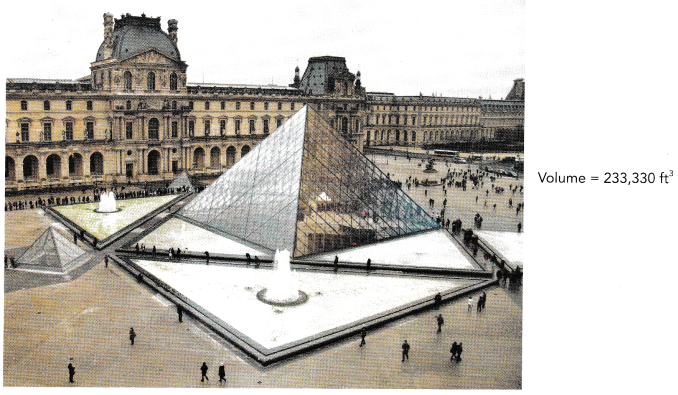Answer: the area of the base of the Square pyramid is 10,000 ft².

Explanation:

the area of the base of the Square pyramid A = a²
Given, V = 233,330 ft³ , h = 70 ft
The volume of the Square pyramid is $$\frac{1}{3}$$ a²h
233,330 = $$\frac{1}{3}$$  × 70 × a²
a² = $$\frac{233,330 × 3}{70}$$
a²= 9999.85 ft = 10,000
a = 100 ft

Then, A = 100 × 100 = 10,000 ft²
So, the area of the base of the Square pyramid is 10,000 ft².

Question 17.
Math Journal Cylinder P and cone Q have the same radius and height. The volume of cylinder P is 393 cubic centimeters. Joseph says that the volume of cone Q is 131 cubic centimeters. Explain how Joseph arrived at his answer.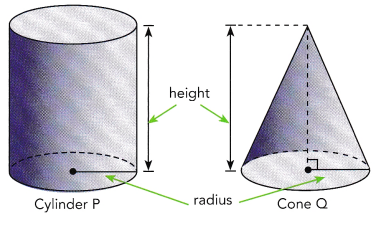Answer:  The volume of the cone and the volume of the cylinder is equal when their respective base and height are equal.

Explanation:
We know that the volume of the cylinder is  V = πr²h
the volume of the cone V=1/3hπr².
Then, The relationship between the volume of a cone and the volume of a cylinder,
with the same base and height is the volume of the cone is one-third the volume of the cylinder.
And, $$\frac{1}{3}$$ πr²h = $$\frac{1}{3}$$ πr²h
Hence, The volume of the cone and the volume of the cylinder is equal when their respective base and height are equal.

Question 18.
A circle has a radius of 61 millimeters. Three-quarters of the circle is used to form a net for the curved part of a cone. The net is taped together to form the cone without any overlap. The height of the finished cone is 40 millimeters.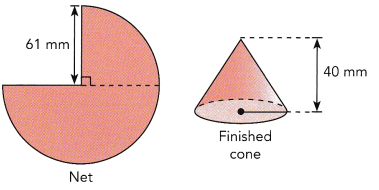a) What is the circumference of the base of the cone?
Answer: The circumference of the base of the cone is  383.08 mm

Explanation:
The circumference of the cone is 2πr
Here d = 61 mm,
Then r = 30.5 mm
C = 2 × 3.14 × 30.5
= 191.54 mm
So, The circumference of the base of the cone is  191.54 mm

b) What is the radius of the cone?

Explanation:
The circumference of the cone is 2πr
Here d = 61 mm,
Then r = 30.5 mm

c) What is the volume of the cone?
Answer: The volume of the cone is 38,946 mm³

Explanation:
the volume of the cone V=1/3hπr².
Here , r = 30.5 mm , h = 40 mm.
V = $$\frac{1}{3}$$ 40 × 30.5 × 30.5 × 3.14
V = 38,946 mm³.
So, The volume of the cone is 38,946 mm³

d) What is the area of the curved surface of the cone?
Answer: The area of the cone’s curved surface is 3,830 mm²

Explanation:
The curved surface area of a cone  S =  πrl
Here r =  30.5 mm , l = 40 mm
S = 3.14 × 30.5 × 40
S = 3,830 mm²
So, The area of the cone’s curved surface is 3,830 mm²

Question 19.
Martha used a filter in the shape of a cone to filter sand from a liquid. The volume of liquid that the filter can hold is 66 cubic centimeters. The height of the filter is 6 centimeters. What is the diameter of the filter? Round your answer to the nearest tenth.
Answer: The diameter of the filter is  7 cm.

Explanation:
Given, V = 66 cm³
the volume of the cone V=1/3hπr².
r = ? , h = 6 cm.
66 = $$\frac{1}{3}$$ 6 × r² × 3.14
r² = $$\frac{66 × 3}{6 × 3.14}$$
r² =10.5 cm
r = 3.24 cm
The radius of the filter  is 3.24 cm

Then, the diameter d = 2r = 2 × 3.24 = 6.48 cm = 7 cm
So, The diameter of the filter is  7 cm.